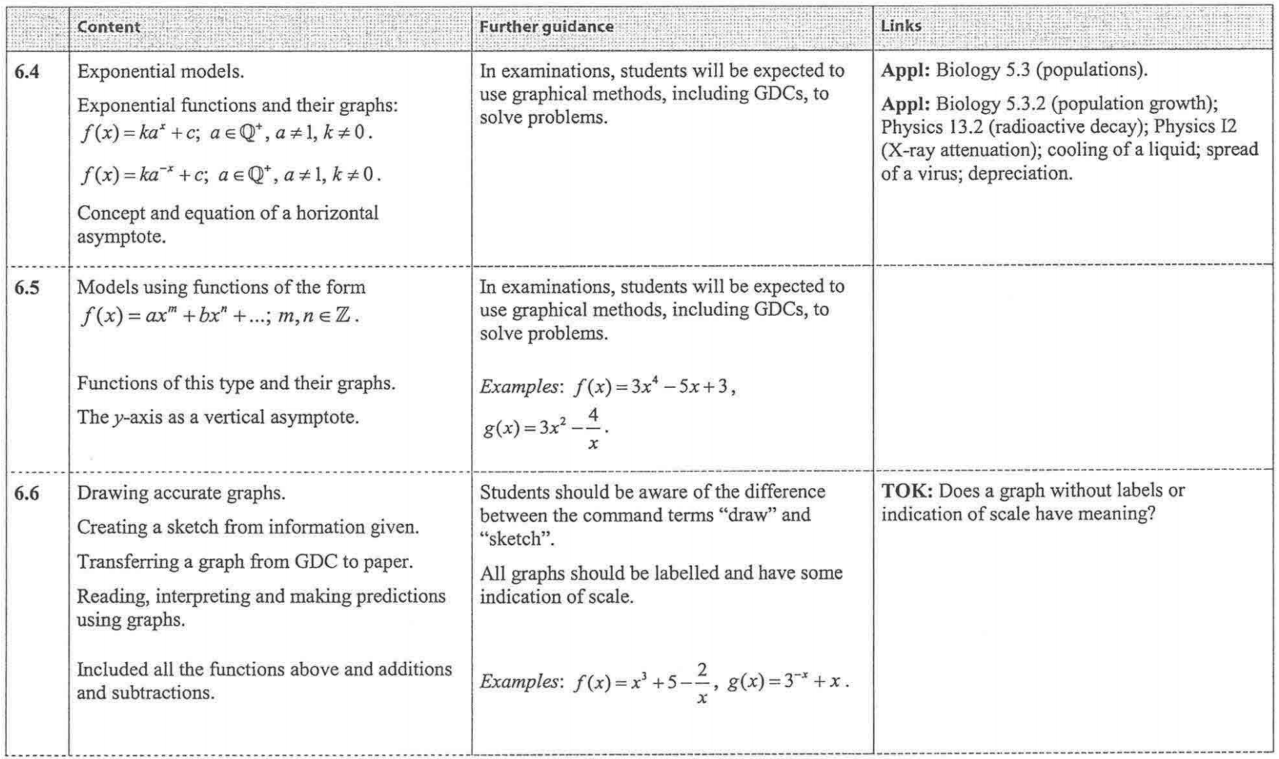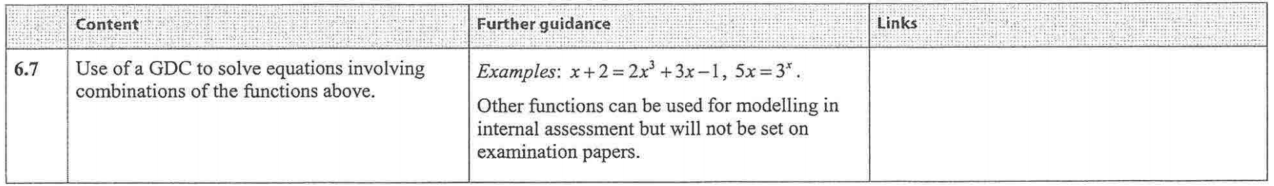### 10. Exponential & Unfamiliar Functions

 IBO Syllabus Topic 6 - Mathematical ModelsTextbook ReferenceChapter 4 p166-1994.4  Exponential models p166-174- Exponential functions and their graphs-Graphs of exponential functions- Applications of exponential functions4.5 Graphs of Polynomial functions p175-187- Cubic functions- Graphs of functions when the power of x in a positive integer- Graphs of functions when the power of x is a negative integer- Graphs of more complex functions- Sketching more complex graphs 4.6 Using a GDC to solve equations p187-1884.7 Graphs of real-life situations p189-192Review exercises p192-197Chapter 2 Summary p198-19910. Exponential and Unfamiliar Function Practice Exam Questions